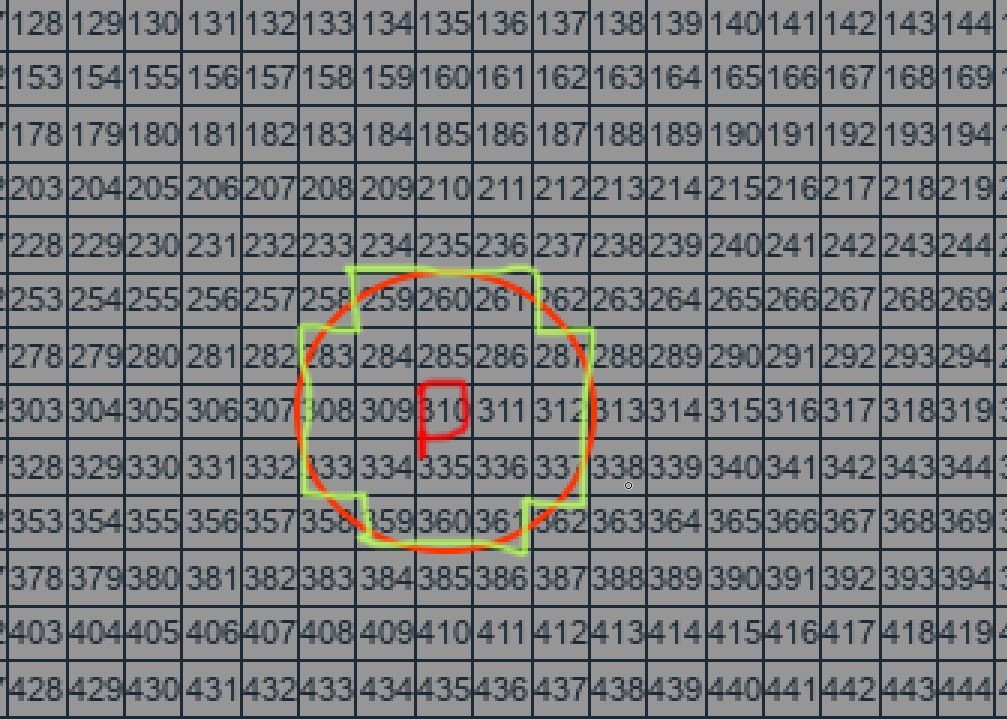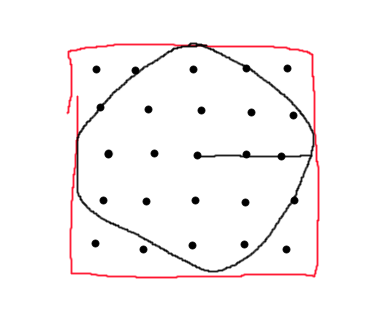# Trigonometry help

On a canvas I want to make the player to be able to paint many pixels at once with a magnitude from the current pixel the player is pointing at.

For context each pixel is indexed by y * total x + x, with y ranging from 0-24 and x ranging from 0-24 as well.
Here’s an image of the pixels with their index overlaid on topHow could I use trigonometry functions such as sine to select indexes that are within a certain radius of the pixel the player is pointed at?

Assume that the filled pixels must all be within a square that encloses the circle. Loop over these pixels and only fill them if their distance from the origin is less than the radius.To clarify any pixel that is mostly covered by the circle will be painted, so it’s necesarry to use trigonometry

You can still just calculate the 2D distance to the center from the midpoint of each square and then if it is within that distance (or equal to) then count that square.

Convert to cartesian coordinates (and back) so you can work with (x, y) coordinates, then do something like

``````local indices = {}
for dx = -r, r do for dy = -r, r do
local d = Vector2.new(dx, dy)
if d.Magnitude > r then continue end
local p = o + d
table.insert(indices, coordinatesToIndex(p.X, p.Y))
end end
``````

There are also more elegant algorithms that calculate how many pixels are in each row based on the height and sin/cos and stuff, but that involves sin and cos and stuff so it’s probably not that much faster.

1 Like
``````--[[ Get distance from object to object in X, Z.
Same as magnitude but in 2 planes ]]
local function getDistance(a, b):
return math.sqrt(((b.X - a.X)^2) + ((b.Y - a.Y)^2))
end

local distance = getDistance(origin.Position, target.Position)
``````

Alright, I’ll try that.

I also already got index to cords and cords to index functions

``````local function ToIndex(x,y)
return y * dX + x
end

local function ToCords(index)
local y = math.floor(index/dX)
local x = index - y * dX
return {x=x,y=y}
end

``````

I’ve made a function for getting valid surrounding pixels, yet it still returns nothing when the index `at` is a valid index of a pixel:

``````local function InRad(at,rad): {Frame}?
if pixels[at] then
local atcords = ToCords(at)
local refsize = pixels[at].Size.X
local listed = {}
local chosen = ToIndex(x,y)
if pixels[chosen] then
local p = pixels[chosen]
local mag = Vector2.new(p.Size.X.Scale,p.Size.Y.Scale).Magnitude / refsize
table.insert(listed,chosen)
end

end
end
end
return listed
else
return
end
end
``````
1 Like

I can’t spot anything obviously wrong. Are you calling it with a radius of more than 1?

Try using the debugger to 'de the bug

With testing I’ve inputted the radius of 3 and with a valid at index.

Edit: turns out that the at index was a wrong value, I’ve got a variable that holds what frame the mouse is currently hovering over, and to get the index i just use the name property of that frame, since its the same.

For whatever reason this didn’t work, so I’ve had setup a variable holding the index of the current frame, which fixed the issue

1 Like

This topic was automatically closed 14 days after the last reply. New replies are no longer allowed.